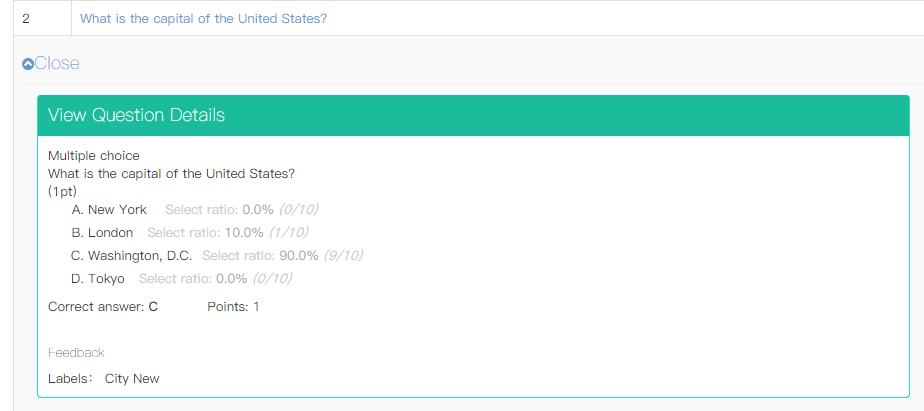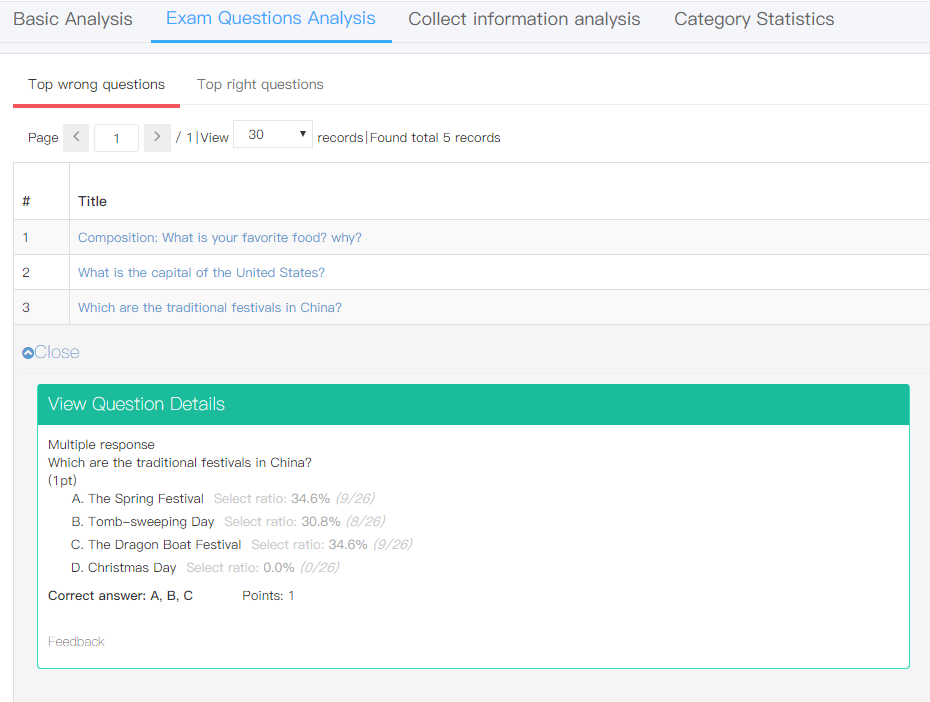# Import questions & manage

## Exam System Calculation on Select Ratio for Choice Questions

How does our Online exam system calculate the select ratio for the type of choice questions?
The illustration is divided in 2 parts, single choice and multiple choice.

1.Single choice question
Formula: Select Ratio = selected times for this option / total taken times for this question
For example: Please refer to the question in the picture below:In this question, option B has been selected for 1 time, option C has been selected for 9 times. And this question has been answered for 10 times. The select ratio for option B is 10%. The select ratio for option C is 90%.

2.Multiple choice question
Differs from the single choice question, the select ratio is relative to the total selected times for all the options.
Formula: Select Ratio = selected times for this option / total selected times for all options
When you enter the exam questions analysis, the select ratio will be shown in the question for your reference:In order to make you more clear about it, please check an example illustration:
For example:
In a multiple choice question, there are 4 choices.
Total 3 candidates have answered this question. Tony has selected options ABC. Tom has selected BCD. Amy has selected BC. So the options they have selected totally are 8 ( =3+3+2).

Option A has been selected for 1 time.
Select ratio for A = 1/8 = 12.5%
Option B has been selected for 3 times.
Select ratio for B = 3/8 = 37.5%
Option C has been selected for 3 times.
Select ratio for C = 3/8 = 37.5%
Option D has been selected for 1 time.
Select ratio for D = 1/8 = 12.5%

Hope this passage is understandable. If you still have any questions, you can sign up and leave us a comment. We are happy to solve problem for you.